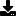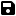Don't have an account yet? Register

# a An Analog Clock which is VERY SIMPLE

by K. O. Thaha Hussain (5 Submissions)
Category: Math/Dates
Compatability: Visual Basic 3.0
Difficulty: Beginner
Date Added: Wed 3rd February 2021
Rating:(30 Votes)Thaha Hussain's Clock-Work Formula:
In most of the computer languages, X-axis is taken correctly. But Y-axis in the reverse of the normal Cartisian axis (Y-axis is incremented downword not upword) . So usual analytic manipulations such as, shifting the origin, calculation of polar co-ordinates etc become difficult. Ofcourse, VB has techniques to correct this problem by scaling. But for many lanuages, it is not available! The formula described can be used in any programming language which has inverted Y-axis (C, BASIC etc.).
These are the difficulties faced while deriving the formulae.
1) The Y axis Problem.
2) Polar angles are measured in anti-clockwise direction, while the clock hands are moved in clockwise direction.
3) 'Zero' of polar angle and 'Zero' of Clock Hands causes a difference of 90 degrees.
The following are the Formulae obtained.
Feel free to use the formula for non-commercial purposes. However the Author's name should be accompanied along with the formula.
Thaha Hussain's clock work formula
Hour Hand :
hour_x2 = LengthOfHourHand * Cos(PI/180*(30 * hour - 90)) + midx
hour_y2 = LengthOfHourHand * Sin(PI/180*(30 * hour - 90)) + midy
Minute Hand:
minute_x2 = LengthOfMinuteHand * Cos(PI/180*(6 * minute - 90)) + midx
minute_y2 = LengthOfMinuteHand * Sin(PI/180*(6 * minute - 90)) + midy
Soconds Hand:
second_x2 = LengthOfSecondsHand * Cos(PI/180*(6 * second - 90)) + midx
second_y2 = LengthOfSecondsHand * Sin(PI/180*(6 * second - 90)) + midy
You'll never get a formula simpler than this to move the clock hands!
1) You can use the formulae in any Programming Language without the Co-ordinate adjustment!
2) No problem for hour, even in 24 Hr format!
3) You can adjust the Length of Clock Hands!
4) Shift the clock to anywhere by changing (midx,midy)
5) Create your own custom controls!
6) A variety of other uses in graphics!
Simply Excellent! Right? Please vote for the formulae!

Assumes
In a form place 3 lines (Line1, Line2, Line3) and a timer (Timer1) and a shape (Shape1). Paste the code.

Rate a An Analog Clock which is VERY SIMPLE

`' In the name of The Almighty...' (C) K. O. Thaha Hussain, Systems Analyst' www.microcentergulf.com Manama, Bahrain' *** LICENSE AGREEMENT ***' Get permission from the author to use' the formulae commercially.' Feel free to make use of the Formulae' for Non-Commercial Purposes,' but the name of the Author should be a' ccompanied along with the formulae.Option ExplicitDim HourLength As Integer, MinuteLength As Integer, _              SecondLength As IntegerDim MidX As Integer, MidY As IntegerConst PI = 3.14159Sub LengthAndCentre()  Dim d As Integer  If Me.ScaleWidth < Me.ScaleHeight Then    HourLength = Me.ScaleWidth * 50 / 200 ' 50%    MinuteLength = Me.ScaleWidth * 80 / 200 ' 80%    SecondLength = Me.ScaleWidth * 90 / 200 ' 90%  Else    HourLength = Me.ScaleHeight * 50 / 200 ' 50%    MinuteLength = Me.ScaleHeight * 80 / 200 ' 80%    SecondLength = Me.ScaleHeight * 90 / 200 ' 90%  End If  MidX = Me.ScaleWidth \ 2  MidY = Me.ScaleHeight \ 2  Line1.X1 = MidX  Line2.X1 = MidX  Line3.X1 = MidX  '  Line1.Y1 = MidY  Line2.Y1 = MidY  Line3.Y1 = MidY  d = Shape1.BorderWidth \ 2  Shape1.Left = d  Shape1.Top = d  Shape1.Width = Me.ScaleWidth - d * 2  Shape1.Height = Me.ScaleHeight - d * 2  Call Timer1_Timer 'just To avoid flickerEnd SubPrivate Sub DrawDial() ' Procedure to draw the dial ' using Clock Work Formula. Dim I, HourX, HourY, MinuteX, MinuteY, DialLength As Integer Me.Cls If Me.ScaleWidth < Me.ScaleHeight Then  DialLength = Me.ScaleWidth * 92 / 200 ' 92% Else  DialLength = Me.ScaleHeight * 92 / 200 ' 92% End If  'The following loop is doing hour marking For I = 1 To 12  Me.DrawWidth = 4  HourX = DialLength * Cos(PI / 180 * (30 * I - 90)) + MidX  HourY = DialLength * Sin(PI / 180 * (30 * I - 90)) + MidY  PSet (HourX, HourY) Next I'The following loop is doing minute marking For I = 1 To 59  Me.DrawWidth = 2  MinuteX = DialLength * Cos(PI / 180 * (6 * I - 90)) + MidX  MinuteY = DialLength * Sin(PI / 180 * (6 * I - 90)) + MidY  PSet (MinuteX, MinuteY) Next IEnd SubPrivate Sub Form_Load()  Me.Caption = "Thaha Hussain's Clock Work Formula"  Me.AutoRedraw = True  Me.BackColor = &H80FF&  '  Shape1.BorderWidth = 4  Shape1.BorderColor = vbYellow  Line1.BorderWidth = 5  Line2.BorderWidth = 3  Line3.BorderWidth = 1  Line3.BorderColor = vbRed  '  Timer1.Interval = 1000  '  Call LengthAndCentre  Call Timer1_Timer 'just To avoid initial flicker  '  MsgBox "Resize the window to resize the clock...", , _  "Thaha Hussain's Clock-Work Formula"End SubPrivate Sub Form_Resize()  On Error Resume Next  Call LengthAndCentre  Call DrawDialEnd SubPrivate Sub Timer1_Timer()  Dim Hours As Single, Minutes As Single, Seconds As Single  Dim TrueHours As Single    'Beep    Hours = Hour(Time)  Minutes = Minute(Time)  Seconds = Second(Time)  TrueHours = Hours + Minutes / 60    'HourHand  Line1.X2 = HourLength * Cos(PI / 180 * (30 * TrueHours - 90)) + MidX  Line1.Y2 = HourLength * Sin(PI / 180 * (30 * TrueHours - 90)) + MidY    'MinuteHand  Line2.X2 = MinuteLength * Cos(PI / 180 * (6 * Minutes - 90)) + MidX  Line2.Y2 = MinuteLength * Sin(PI / 180 * (6 * Minutes - 90)) + MidY    'SecondsHand  Line3.X2 = SecondLength * Cos(PI / 180 * (6 * Seconds - 90)) + MidX  Line3.Y2 = SecondLength * Sin(PI / 180 * (6 * Seconds - 90)) + MidYEnd Sub`Download this snippetAdd to My Saved Code